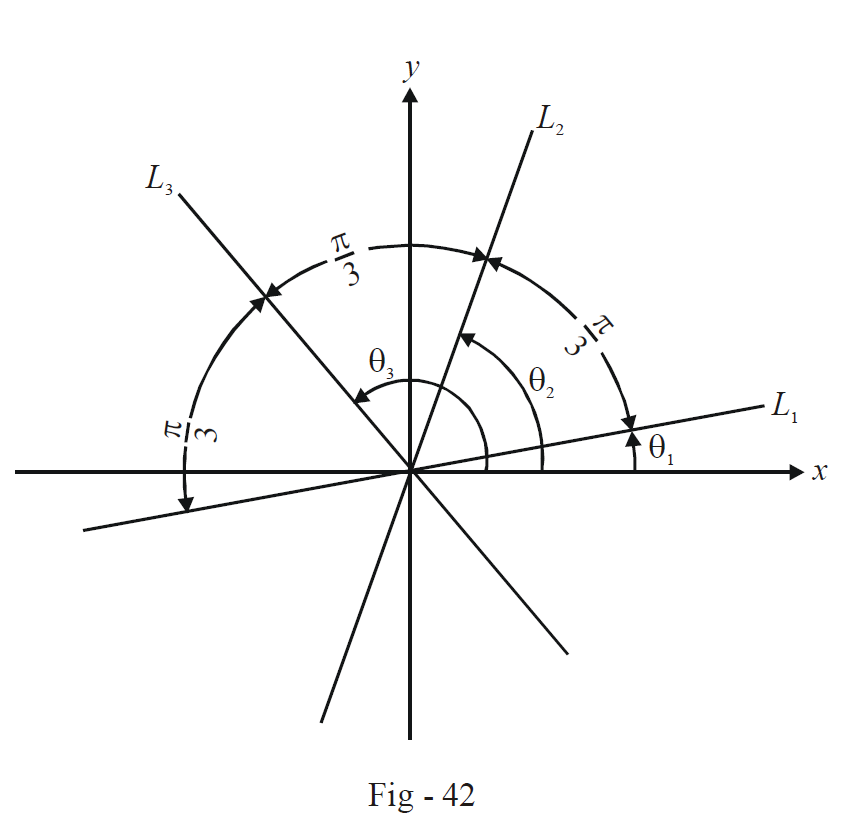# Straight Lines Set 5

Go back to  'Straight Lines'

Example – 9

Show that the equation

$\lambda ({x^3} - 3x{y^2}) + {y^3} - 3{x^2}y = 0$

represents three straight lines equally inclined to one another.

Solution: Observe that since the equation is homogenous, it will represent three straight lines passing through the origin. Let the slopes of the three lines be $${m_1},\,{m_2}$$ and $${m_3}.$$

Thus $${m_1},\,{m_2}$$ and $${m_3}$$ are the roots of the equation

\begin{align}&\lambda (1 - 3{m^2}) + {m^3} - 3m = 0\,\,\,\,\,\,\,\,\,\,\,\,\,\,\,\left( {{\text{where }}m = \frac{y}{x}} \right) \\ \Rightarrow \qquad & \frac{{3m - {m^3}}}{{1 - 3{m^2}}} = \lambda \\ \end{align}

Since $$m = \frac{y}{x} = \tan \theta ,$$ where $$\theta$$ is the inclination of the line, we have

\begin{align}&\lambda = \frac{{3\tan \theta - {{\tan }^3}\theta }}{{1 - 3{{\tan }^2}\theta }} = \tan 3\theta \\ \Rightarrow \qquad& \tan 3\theta = \lambda \\ \Rightarrow \qquad& 3\theta = n\pi + {\tan ^{ - 1}}\lambda \\ \Rightarrow \qquad& \theta = \frac{{n\pi + {{\tan }^{ - 1}}\lambda }}{3}\\ \end{align}

Since there are three lines corresponding to the joint equation, we’ll have three corresponding angles of inclination

${\theta _1} = \frac{{{{\tan }^{ - 1}}\lambda }}{3}, \; {\theta _2} = \frac{\pi }{3} + {\tan ^{ - 1}}\lambda ,\,\,\,\,\,{\theta _3} = \frac{{2\pi }}{3} + {\tan ^{ - 1}}\lambda$

The angles of inclination show that the three lines are equally inclined to one another.Example –10

Show that all the chords of the curve  $$3{x^2} - {y^2} - 2x + 4y = 0$$  which subtend a right angle at the origin pass through a fixed point. Find that point.

Solution: Let $$y = mx + c$$ be a chord of the curve which subtends a right angle at the origin. The joint equation of the lines joining the origin to points of intersection of  $$y = mx + c$$ and the curve is

$3{x^2} - {y^2} + (4y - 2x)\left( {\frac{{y - mx}}{c}} \right) = 0$

This represents two perpendicular lines if

\begin{align}&{\text{Coeff}}{\text{. of }}{x^2} + {\text{Coeff}}{\text{. of }}{y^2} = 0 \\ &3 + \frac{{2m}}{c} + \frac{4}{c} - 1 = 0 \\ \Rightarrow \qquad& c + m + 2 = 0 \\ \Rightarrow \qquad& ( - 2) = m(1) + c\,\,\,\,\,\,\,\,\,\,\,\,\,\,\,\,\,\,\,\,\,\,\,\,\,\,\,\, \ldots \left( 1 \right) \\ \end{align}

(1) shows that $$y = mx + c$$ always passes through the fixed point (–2, 1).